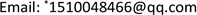﻿ 波动方程：拟稳定性和广义指数吸引子 Wave Equation: Quasi-Stability and Generalized Exponential Attractor

Pure Mathematics
Vol. 08  No. 05 ( 2018 ), Article ID: 26757 , 8 pages
10.12677/PM.2018.85068

Wave Equation: Quasi-Stability and Generalized Exponential Attractor

Na Fu*, Linlin Liu

School of Mathematics, Southwest Jiaotong University, Chengdu SichuanReceived: Aug. 19th, 2018; accepted: Sep. 4th, 2018; published: Sep. 11th, 2018ABSTRACT

This paper studies the long-term dynamical behavior of the solution of the wave equation. By using the methods developed by Chueshov and Lasiecha, we get the quasi-stability property of the system and obtain the existence of a global attractor which has finite fractal dimension. Result on exponential attractors of the system is also proved.

Keywords:Wave Equation, Quasi-Stability, Global Attractor, Fractal Dimension, Exponential Attractor1. 引言

$\left\{\begin{array}{l}{u}_{tt}+\alpha {u}_{t}-\Delta u+f\left(u\right)=q\left(x\right),\text{ }\text{ }\text{ }\text{ }\text{ }\text{ }\text{ }\text{ }\text{ }\text{ }\text{ }\text{ }\text{ }\text{ }\text{ }\text{ }\text{ }\text{ }\text{in}\text{\hspace{0.17em}}\text{ }\Omega ,t>0,\\ u|{}_{\Gamma }={u}_{t}|{}_{\Gamma }=0,\text{ }\text{ }\text{ }\text{ }\text{ }\text{ }\text{ }\text{ }\text{ }\text{ }\text{ }\text{ }\text{ }\text{ }\text{ }\text{ }\text{ }\text{ }\text{ }\text{ }\text{ }\text{ }\text{ }\text{ }\text{ }\text{ }\text{ }\text{ }\text{ }\text{ }\text{ }\text{ }\text{ }\text{ }\text{ }\text{ }\text{ }\text{ }\text{ }\text{ }\text{ }\text{ }\text{ }\text{ }\text{ }\text{ }\text{ }\text{ }\text{ }\text{ }\text{ }\text{ }\text{ }\text{ }\text{ }\text{ }\text{ }\text{ }\text{ }\text{ }\text{ }\text{ }\text{ }\text{ }\text{ }\text{ }\text{ }\text{ }\text{ }\text{ }\text{ }\text{ }\text{ }\text{ }\text{ }\text{ }\text{on}\text{ }\text{\hspace{0.17em}}\Omega ,t>0,\\ u\left(0,x\right)={u}_{0}\left(x\right)\in {H}_{0}^{1}\left(\Omega \right),\\ {u}_{t}\left(0,x\right)={u}_{1}\left(x\right)\in {L}^{2}\left(\Omega \right),\end{array}$ (1)

$\underset{|s|\to \infty }{\mathrm{lim}\mathrm{inf}}\frac{F\left(s\right)}{{s}^{2}}\ge 0，$ (2)

$\underset{|s|\to \infty }{\mathrm{lim}\mathrm{inf}}\frac{sf\left(s\right)-{c}_{1}F\left(s\right)}{{s}^{2}}\ge 0，$ (3)

$|{f}^{\prime }\left(s\right)|\le {c}_{2}\left(1+{|s|}^{2}\right)，$ (4)

2. 半群

${\left(u,v\right)}_{0}={\int }_{\Omega }uv\text{d}x$${‖u‖}_{0}^{2}={\left(u,u\right)}_{0}$

${\left(u,v\right)}_{1}={\left(u,v\right)}_{0}+{\left(\nabla u,\nabla v\right)}_{0}$${‖u‖}_{1}^{2}={\left(u,u\right)}_{1}$

${\left(w,{w}^{\prime }\right)}_{H}={\left({w}_{1},{{w}^{\prime }}_{1}\right)}_{1}+{\left({w}_{2},{{w}^{\prime }}_{2}\right)}_{0}$${‖w‖}_{H}^{2}={‖{w}_{1}‖}_{1}^{2}+{‖{w}_{2}‖}_{0}^{2}$

$D\left(A\right)=\left\{u,-\Delta u\in {L}^{2}\left(\Omega \right),u|{}_{\Gamma }={u}_{t}|{}_{\Gamma }=0\right\}$

A的特征值为 ${\left\{{\lambda }_{i}\right\}}_{i\in {N}^{+}}$ 满足 $0\le {\lambda }_{1}\le {\lambda }_{2}\le \cdots \le {\lambda }_{m}\le \cdots$ ，且当 $m\to \infty$${\lambda }_{m}\to \infty$

$\left\{\begin{array}{l}\frac{\text{d}u}{\text{d}t}=v,\\ \frac{\text{d}v}{\text{d}t}+\alpha v-\Delta u+f\left(u\right)=q\left(x\right),\\ u|{}_{\Gamma }=v|{}_{\Gamma }=0,\\ u\left(0,x\right)={u}_{0}\left(x\right)\in {H}_{0}^{1}\left(\Omega \right),\\ v\left(0,x\right)={u}_{1}\left(x\right)\in {L}^{2}\left(\Omega \right),\end{array}$ (5)

$W=\left[\begin{array}{c}u\\ v\end{array}\right]$$L=\left[\begin{array}{c}-v\\ \alpha v-\Delta u\end{array}\right]$$G\left(W\right)=\left[\begin{array}{c}0\\ q\left(x\right)-f\left(u\right)\end{array}\right]$

$\frac{\text{d}W}{\text{d}t}+LW=G\left(W\right)$$W\left(0\right)={W}_{0}$(6)

$W\left(t\right)={\text{e}}^{-Lt}W\left(0\right)+{\int }_{0}^{\infty }{\text{e}}^{-L\left(t-s\right)}G\left(W\left(s\right)\right)\text{d}s,\text{\hspace{0.17em}}t\ge 0$

$T\left(t\right):W\left(0\right)={\left({u}_{0},{u}_{1}\right)}^{T}\to W\left(t\right)={\left(u\left(t\right),{u}_{t}\left(t\right)\right)}^{T},\text{ }\text{ }\text{ }\text{ }\text{ }\text{ }\text{ }\text{ }\text{ }H\to H.$

3. 吸收集

${\phi }_{1}=u,{\phi }_{2}={u}_{t}+\epsilon u$ ，其中 $\epsilon =\frac{\alpha \left({\alpha }^{2}-1\right)}{3\left({\alpha }^{2}-1\right)+\sqrt{{\alpha }^{4}-1}}$ ，因此方程(1)可写作如下形式

$\left\{\begin{array}{l}\frac{\text{d}{\phi }_{1}}{\text{d}t}+\epsilon {\phi }_{1}-{\phi }_{2}=0,\\ \frac{\text{d}{\phi }_{2}}{\text{d}t}-\epsilon {\phi }_{2}+{\epsilon }^{2}{\phi }_{1}+\alpha \left({\phi }_{2}-\epsilon {\phi }_{1}\right)-\Delta {\phi }_{1}-f\left({\phi }_{1}\right)=q\left(x\right),\\ u|{}_{\Gamma }=v|{}_{\Gamma }=0,\\ u\left(0,x\right)={u}_{0}\left(x\right)\in {H}_{0}^{1}\left(\Omega \right),\\ v\left(0,x\right)={u}_{1}\left(x\right)\in {L}^{2}\left(\Omega \right),\end{array}$ (7)

$\theta =\left[\begin{array}{c}{\phi }_{1}\\ {\phi }_{2}\end{array}\right]$${L}_{\epsilon }\left(\theta \right)=\left[\begin{array}{c}\epsilon {\phi }_{1}-{\phi }_{2}\\ -\epsilon {\phi }_{2}+{\epsilon }^{2}{\phi }_{1}+\alpha \left({\phi }_{2}-\epsilon {\phi }_{1}\right)-\Delta {\phi }_{1}\end{array}\right]$$G\left(\theta \right)=\left[\begin{array}{c}0\\ q\left(x\right)-f\left({\phi }_{1}\right)\end{array}\right]$

$\frac{\text{d}\theta }{\text{d}t}+{L}_{\epsilon }\left(\theta \right)=G\left(\theta \right)$$\theta \left(0\right)={\left({\phi }_{1}\left(0\right),{\phi }_{2}\left(0\right)\right)}^{\text{T}}$(8)

${\left({L}_{\epsilon }\left(\theta \right),\theta \right)}_{H}\ge \frac{\epsilon }{2}{‖\theta ‖}_{H}^{2}+\frac{\alpha }{2}{‖{\phi }_{2}‖}_{0}^{2}$

${\left({L}_{\epsilon }\left(\theta \right),\theta \right)}_{H}={\left(\epsilon {\phi }_{1}-{\phi }_{2},{\phi }_{1}\right)}_{1}+{\left(-\epsilon {\phi }_{2}+{\epsilon }^{2}{\phi }_{1}+\alpha \left({\phi }_{2}-\epsilon {\phi }_{1}\right)-\Delta {\phi }_{1},{\phi }_{2}\right)}_{0}$

$\begin{array}{l}{\left({L}_{\epsilon }\left(\theta \right),\theta \right)}_{H}-\frac{\epsilon }{2}{‖\theta ‖}_{H}^{2}-\frac{\alpha }{2}{‖{\phi }_{2}‖}_{0}^{2}\\ =\epsilon {‖{\phi }_{1}‖}_{1}^{2}-{\left({\phi }_{1},{\phi }_{2}\right)}_{1}+\left({\epsilon }^{2}-\alpha \epsilon \right){\left({\phi }_{1},{\phi }_{2}\right)}_{0}-\epsilon {‖{\phi }_{2}‖}_{0}^{2}+\alpha {‖{\phi }_{2}‖}_{0}^{2}\\ \text{\hspace{0.17em}}\text{\hspace{0.17em}}\text{ }\text{ }-{\left(\Delta {\phi }_{1},{\phi }_{2}\right)}_{0}-\frac{\epsilon }{2}{‖{\phi }_{1}‖}_{1}^{2}-\frac{\epsilon }{2}{‖{\phi }_{2}‖}_{0}^{2}-\frac{\alpha }{2}{‖{\phi }_{2}‖}_{0}^{2}\\ \ge \frac{\epsilon }{2}{‖{\phi }_{1}‖}_{0}^{2}-\left(1-{\epsilon }^{2}+\alpha \epsilon \right){‖{\phi }_{1}‖}_{0}{‖{\phi }_{2}‖}_{0}+\left(\frac{\alpha }{2}-\frac{3\epsilon }{2}\right){‖{\phi }_{2}‖}_{0}^{2}\end{array}$

$\alpha -3\epsilon \ge 0$$2\sqrt{\frac{\epsilon }{2}\cdot \frac{\alpha -3\epsilon }{2}}\ge 1-{\epsilon }^{2}+\alpha \epsilon$

${‖{T}_{\epsilon }\left(t\right)\phi \left(0\right)‖}_{H}^{2}\le {C}_{1}$

$\frac{1}{2}\frac{\text{d}}{\text{d}t}{‖\theta ‖}_{H}^{2}+\frac{\epsilon }{2}{‖\theta ‖}_{H}^{2}+\frac{\alpha }{2}{‖{\phi }_{2}‖}_{0}^{2}+{\left(F\left({\phi }_{1}\right),1\right)}_{0}+{\left(f\left({\phi }_{1}\right),{\phi }_{1}\right)}_{0}\le {\left(q\left(x\right),{\phi }_{2}\right)}_{0}$

${\left({\phi }_{1},f\left({\phi }_{1}\right)\right)}_{0}\ge {c}_{1}{\left(F\left({\phi }_{1}\right),1\right)}_{0}-\frac{1}{16}{‖\phi ‖}_{1}^{2}-k$(9)

${\left(F\left({\phi }_{1}\right),1\right)}_{0}\ge -\frac{1}{16\left(1+{c}_{1}\right)}{‖\phi ‖}_{1}^{2}-k$(10)

$\frac{1}{2}\frac{\text{d}}{\text{d}t}\left({‖\theta ‖}_{H}^{2}+2{\left(F\left({\phi }_{1}\right),1\right)}_{0}\right)+\frac{7\epsilon }{16}{‖{\phi }_{1}‖}_{1}^{2}+\left(\frac{\epsilon }{2}+\frac{\alpha }{2}\right){‖{\phi }_{2}‖}_{0}^{2}+{c}_{1}\epsilon {\left(F\left({\phi }_{1}\right),1\right)}_{0}\le {\left(q,{\phi }_{2}\right)}_{0}+\epsilon k$

$\frac{1}{2}\frac{\text{d}}{\text{d}t}\left({‖\phi ‖}_{H}^{2}+2{\left(F\left({\phi }_{1}\right),1\right)}_{0}\right)+\frac{7\epsilon }{16}{‖{\phi }_{1}‖}_{1}^{2}+\frac{7\epsilon }{16}{‖{\phi }_{2}‖}_{0}^{2}+{c}_{1}\epsilon {\left(F\left({\phi }_{1}\right),1\right)}_{0}\le \frac{1}{2\alpha }{‖q‖}_{0}^{2}+\epsilon k$

$\frac{\text{d}}{\text{d}t}\left({‖\theta ‖}_{H}^{2}+2{\left(F\left({\phi }_{1}\right),1\right)}_{0}\right)+\frac{7\epsilon }{8}\left({‖\theta ‖}_{H}^{2}+2{c}_{1}{\left(F\left({\phi }_{1}\right),1\right)}_{0}\right)\le \frac{1}{\alpha }{‖q‖}_{0}^{2}+2\epsilon k$

${‖\theta ‖}_{H}^{2}+2{\left(F\left({\phi }_{1}\right),1\right)}_{0}\ge \frac{7}{8}{‖\theta ‖}_{H}^{2}$

${‖\theta ‖}_{H}^{2}+2{\left(F\left({\phi }_{1}\right),1\right)}_{0}\le {‖\theta ‖}_{H}^{2}+2{\left(F\left({\phi }_{1}\right),1\right)}_{0}{\text{e}}^{-\frac{7{k}_{1}\epsilon }{8}t}+\left(\frac{8}{7\alpha {k}_{1}\epsilon }{‖q‖}_{0}^{2}+\frac{16k}{7{k}_{1}}\right)\left(1-{\text{e}}^{-\frac{7{k}_{1}\epsilon }{8}t}\right)$

${k}_{1}=\mathrm{min}\left\{1,4{c}_{1}\right\}$

${‖{\theta }_{0}‖}_{H}^{2}\le r$ ，由(4)和Sobolev’s嵌入定理，则存在 $K\left(r\right)>0$ ，使得

${‖\theta ‖}_{H}^{2}+2{\left(F\left({\phi }_{1}\right),1\right)}_{0}\le K\left(r\right)$

${‖{\theta }_{0}‖}_{H}^{2}\le \frac{8}{7}K\left(r\right){\text{e}}^{-\frac{{k}_{1}\epsilon }{4}t}+\left(\frac{64}{49\alpha {k}_{1}\epsilon }{‖q‖}_{0}^{2}+\frac{128k}{49{k}_{1}}\right)\left(1-{\text{e}}^{-\frac{{k}_{1}\epsilon }{4}t}\right)$

${C}_{1}=\frac{64}{49\alpha {k}_{1}\epsilon }{‖q‖}_{0}^{2}+\frac{128k}{49{k}_{1}}$

${T}_{\epsilon }\left(t\right){B}_{0}\subseteq {B}_{0}$

4. 动力系统的拟稳定性和渐进光滑性

$S\left(t\right){U}_{0}=\left(u,{u}_{t}\right)$${U}_{0}=\left(u\left(0\right),{u}_{t}\left(0\right)\right)\in H$(11)

$u\in C\left({R}^{+},X\right)\cap {C}^{1}\left({R}^{+},Y\right)$(12)

(i) $a\left(t\right)$$c\left(t\right)$$\left[0,+\infty \right)$ 上局部有界，

(ii) $b\left(t\right)\in {L}^{1}\left({R}^{+}\right)$${\mathrm{lim}}_{t\to \infty }b\left(t\right)=0$

(iii) 对任意的 ${u}^{1},{u}^{2}\in B$$t>0$ 下面的关系成立：

${‖S\left(t\right){U}^{1}-S\left(t\right){U}^{2}‖}_{H}^{2}\le a\left(t\right){‖{U}^{1}-{U}^{2}‖}_{H}^{2}$(13)

${‖S\left(t\right){U}^{1}-S\left(t\right){U}^{2}‖}_{H}^{2}\le b\left(t\right){‖{U}^{1}-{U}^{2}‖}_{H}^{2}+c\left(t\right)\underset{0(14)

$‖{T}_{\epsilon }{U}^{1}-{T}_{\epsilon }{U}^{2}‖\le {b}_{0}{\text{e}}^{-rt}{‖{U}^{1}-{U}^{2}‖}_{H}^{2}+{C}_{B}\left(1-{\text{e}}^{-rt}\right){‖{u}^{1}-{u}^{2}‖}_{0}^{2}$(15)

$\frac{\text{d}\Phi }{\text{d}t}+{L}_{\epsilon }\left(\Phi \right)=G\left(\Phi \right)$(16)

${U}^{1}\left(0\right)-{U}^{2}\left(0\right)=\left({\Phi }_{0},{\Phi }_{1}\right)$

$\begin{array}{c}\frac{1}{2}\frac{\text{d}}{\text{d}t}{‖\Phi ‖}_{H}^{2}+\frac{\epsilon }{2}{‖\Phi ‖}_{H}^{2}+\frac{\alpha }{2}{‖\Phi ‖}_{0}^{2}\le -{\left(f\left({u}^{1}\right)-f\left({u}^{2}\right),{\Phi }_{2}\right)}_{0}\\ \le {‖f\left({u}^{1}\right)-f\left({u}^{2}\right)‖}_{0}{‖{\Phi }_{2}‖}_{0}\\ \le \frac{1}{2\alpha }{‖f\left({u}^{1}\right)-f\left({u}^{2}\right)‖}_{0}^{2}+\frac{\alpha }{2}{‖{\Phi }_{2}‖}_{0}^{2}\end{array}$

$\begin{array}{c}{‖f\left({u}^{1}\right)-f\left({u}^{2}\right)‖}_{0}^{2}={\int }_{\Omega }{\left(f\left({u}^{1}\right)-f\left({u}^{2}\right)\right)}^{2}\text{d}x\le {C}_{2}{\int }_{\Omega }{\left({|{u}^{1}|}^{3}-{|{u}^{2}|}^{3}\right)}^{2}\text{d}x\\ \le {\left({C}_{2}{\int }_{\Omega }{\left({|{u}^{1}|}^{2}+{u}^{1}{u}^{2}+{|{u}^{2}|}^{2}\right)}^{3}\text{d}x\right)}^{\frac{2}{3}}{\left({\int }_{\Omega }{\left({u}^{1}-{u}^{2}\right)}^{6}\text{d}x\right)}^{\frac{1}{3}}\\ \le {C}_{2}\left(\frac{1}{4k}{‖{|{u}^{1}|}^{2}+{u}^{1}{u}^{2}+{|{u}^{2}|}^{2}‖}_{{L}^{3}}^{2}+k{‖{u}^{1}-{u}^{2}‖}_{{L}^{6}}^{2}\right)\\ \le K{‖{u}^{1}-{u}^{2}‖}_{{L}^{6}}^{2}\le K{‖{u}^{1}-{u}^{2}‖}_{{H}_{0}^{1}}^{2}\end{array}$

$\frac{1}{2}\frac{\text{d}}{\text{d}t}{‖\Phi ‖}_{H}^{2}+\frac{\epsilon }{2}{‖\Phi ‖}_{H}^{2}\le K{‖{u}^{1}-{u}^{2}‖}_{{H}_{0}^{1}}^{2}$

${‖\Phi \left(t\right)‖}_{H}^{2}\le {‖\Phi \left(0\right)‖}_{H}^{2}{\text{e}}^{-\epsilon t}+\frac{2k}{\epsilon }{‖{u}^{1}-{u}^{2}‖}_{{H}_{0}^{1}}^{2}\left(1-{\text{e}}^{-\epsilon t}\right)$

${‖{T}_{\epsilon }{U}^{1}-{T}_{\epsilon }{U}^{2}‖}_{H}^{2}\le {\text{e}}^{-\epsilon t}{‖{U}^{1}-{U}^{2}‖}_{H}^{2}+\frac{2k}{\epsilon }\left(1-{\text{e}}^{-\epsilon t}\right){‖{u}^{1}-{u}^{2}‖}_{{H}_{0}^{1}}^{2}$

$B\subset H$ 关于 $T\left(t\right)$ 的有界正向不变集。设 $T\left(t\right){U}^{i}=\left({u}^{i},{u}^{i}+\epsilon {u}^{i}\right)$ 是方程(8)关于初值条件 ${U}^{i},i=1,2$ 在B中的解，我们定义半范数

${n}_{X}\left(\Phi \right)={‖\Phi ‖}_{{L}^{2}}^{2}$

${‖{T}_{\epsilon }{U}^{1}-{T}_{\epsilon }{U}^{2}‖}_{H}^{2}\le b\left(t\right){‖{U}^{1}-{U}^{2}‖}_{H}^{2}+c\left(t\right)\underset{0

$b\left(t\right)\in {L}^{1}\left({R}^{+}\right)$ 并且 $\underset{t\to \infty }{\mathrm{lim}}b\left(t\right)=0$

5. 全局吸引子和广义指数吸引子

${‖{U}_{t}\left(t\right)‖}_{\stackrel{˜}{H}}\le {C}_{B}$

${‖{T}_{\epsilon }\left({t}_{1}\right)U-{T}_{\epsilon }\left({t}_{2}\right)U‖}_{\stackrel{˜}{H}}\le {\int }_{{t}_{1}}^{{t}_{2}}{‖{U}_{t}\left(\tau \right)‖}_{\stackrel{˜}{H}}\text{d}\tau \le {C}_{B}{|{t}_{1}-{t}_{2}|}^{r},{t}_{1},{t}_{2}\in \left[0,T\right],U\in B$ (17)

Wave Equation: Quasi-Stability and Generalized Exponential Attractor[J]. 理论数学, 2018, 08(05): 508-515. https://doi.org/10.12677/PM.2018.85068

1. 1. Temam, R. (1998) Infinite-Dimension Dynamical Systems in Mechanics and Physics. 2nd Edition, New York.

2. 2. Aviles, P. and Sandefur, J. (1985) Nonlinear Second Order Equations with Applications to Partial Differential Equations. Journal of Differential Equations, 58, 404-427. https://doi.org/10.1016/0022-0396(85)90008-7

3. 3. Fitzgibbon, W.E. (1981) Strongly Damped Quasi-linear Evolution Equations. Journal of Mathematical Analysis & Applications, 79, 536-550.

4. 4. Zelik (1991) Asymptotic Regularity of Solutions of Singularly Perturbed Damped Wave Equations with Supercritical Nonlinearities.

5. 5. Frigeri, S. (2010) Attractors for Semilinear Damped Wave Equations with an Acoustic Boundary Condition. Journal of Evolution Equations, 10, 29-58. https://doi.org/10.1007/s00028-009-0039-1

6. 6. Chueshov, I. and Lasiecka, I. (2012) Von Karman Evolution Equation: Well-Posedness and Longtime Dynamics. Springer, New York.

7. 7. Feng, B. (2017) On a Semilinear Timoshenko-Colean-Gurtin System: Quasi-Stability and Attractors. Discrete Continuous Dynamical Systems, 3, 4427-4451.

8. 8. Beale, J.T. (1967) Spectral Properties of an Acoustic Boundary Conditions. Indiana University Mathematics Journal, 25, 895-917. https://doi.org/10.1512/iumj.1976.25.25071